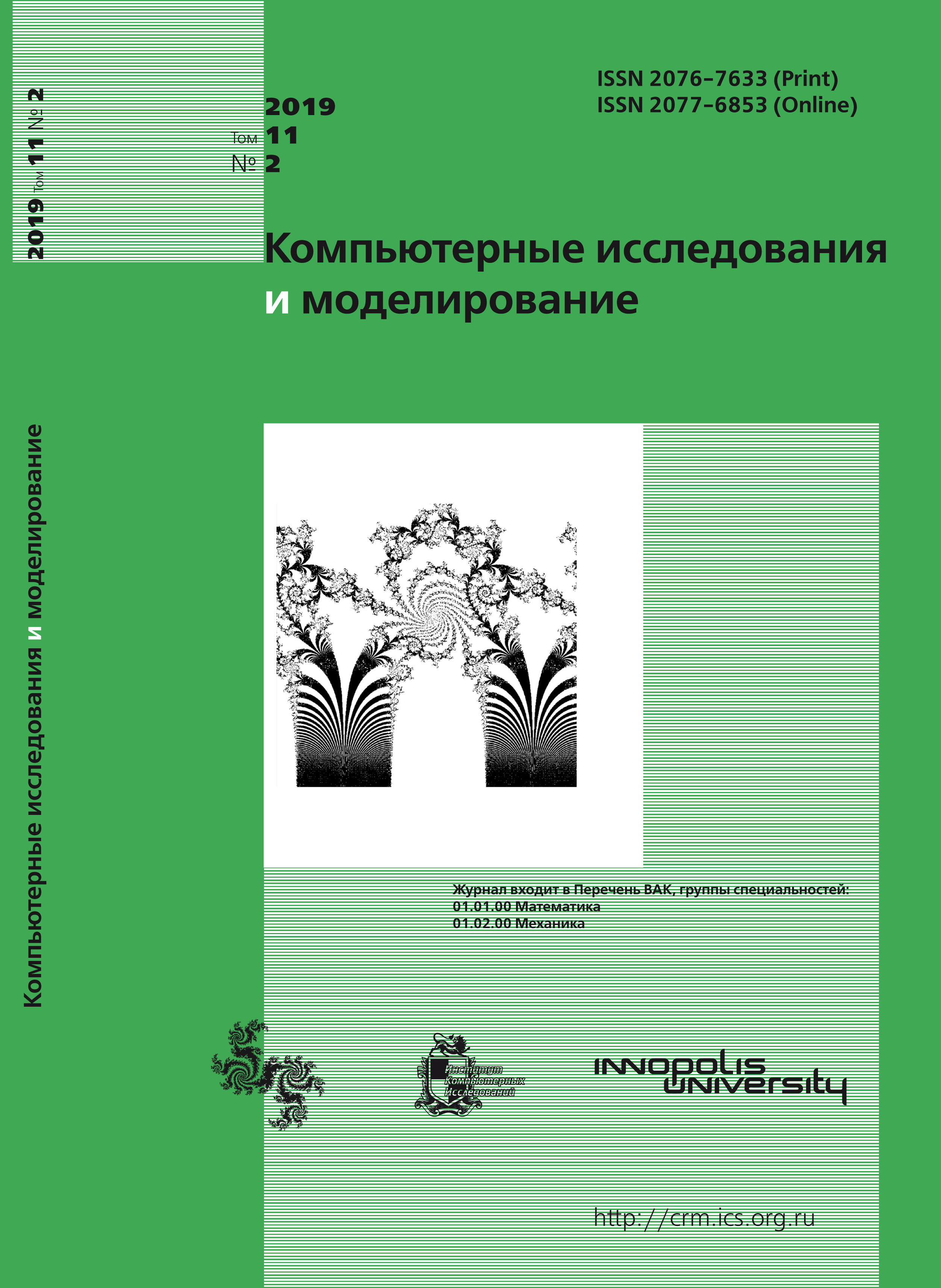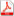Issue 2, 2019 Vol. 11

# All issues

A problem-modeling environment for the numerical solution of the Boltzmann equation on a cluster architecture for analyzing gas-kinetic processes in the interelectrode gap of thermal emission converterspdf (4596K)  / List of references

This paper is devoted to the application of the method of numerical solution of the Boltzmann equation for the solution of the problem of modeling the behavior of radionuclides in the cavity of the interelectric gap of a multielement electrogenerating channel. The analysis of gas-kinetic processes of thermionic converters is important for proving the design of the power-generating channel. The paper reviews two constructive schemes of the channel: with one- and two-way withdrawal of gaseous fission products into a vacuum-cesium system. The analysis uses a two-dimensional transport equation of the second-order accuracy for the solution of the left-hand side and the projection method for solving the right-hand side — the collision integral. In the course of the work, a software package was implemented that makes it possible to calculate on the cluster architecture by using the algorithm of parallelizing the left-hand side of the equation; the paper contains the results of the analysis of the dependence of the calculation efficiency on the number of parallel nodes. The paper contains calculations of data on the distribution of pressures of gaseous fission products in the gap cavity, calculations use various sets of initial pressures and flows; the dependency of the radionuclide pressure in the collector region was determined as a function of cesium pressures at the ends of the gap. The tests in the loop channel of a nuclear reactor confirm the obtained results.

Keywords: rarefied gas, gas mixture, Boltzmann equation, conservative projection method, numerical simulation
Citation in English: Basalaev A.V., Kloss Y.Y., Lubimov D.U., Knyazev A.N., Shuvalov P.V., Sherbakov D.V., Nahapetyan A.V. A problem-modeling environment for the numerical solution of the Boltzmann equation on a cluster architecture for analyzing gas-kinetic processes in the interelectrode gap of thermal emission converters // Computer Research and Modeling, 2019, vol. 11, no. 2, pp. 219-232
Citation in English: Basalaev A.V., Kloss Y.Y., Lubimov D.U., Knyazev A.N., Shuvalov P.V., Sherbakov D.V., Nahapetyan A.V. A problem-modeling environment for the numerical solution of the Boltzmann equation on a cluster architecture for analyzing gas-kinetic processes in the interelectrode gap of thermal emission converters // Computer Research and Modeling, 2019, vol. 11, no. 2, pp. 219-232
DOI: 10.20537/2076-7633-2019-11-2-219-232
Views (last year): 24.

Full-text version of the journal is also available on the web site of the scientific electronic library eLIBRARY.RU

The journal is included in the Russian Science Citation Index

The journal is included in the List of Russian peer-reviewed journals publishing the main research results of PhD and doctoral dissertations.

International Interdisciplinary Conference "Mathematics. Computing. Education"

The journal is included in the RSCI

Indexed in Scopus